# Maxwell's Equations

Maxwell's equations represent one of the most elegant and concise ways to state the fundamentals of electricity and magnetism. From them one can develop most of the working relationships in the field. Because of their concise statement, they embody a high level of mathematical sophistication and are therefore not generally introduced in an introductory treatment of the subject, except perhaps as summary relationships.

These basic equations of electricity and magnetism can be used as a starting point for advanced courses, but are usually first encountered as unifying equations after the study of electrical and magnetic phenomena.

 Symbols Used E = Electric field ρ = charge density i = electric current B = Magnetic field ε0 = permittivity J = current density D = Electric displacement μ0 = permeability c = speed of light H = Magnetic field strength M = Magnetization P = Polarization
 Integral form Differential form
Index

Maxwell's equations concepts

 HyperPhysics***** Electricity and Magnetism R Nave
Go Back

# Maxwell's Equations

Integral form in the absence of magnetic or polarizable media:

 I. Gauss' law for electricity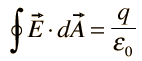II. Gauss' law for magnetism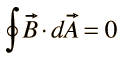III. Faraday's law of induction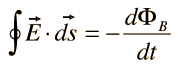IV. Ampere's law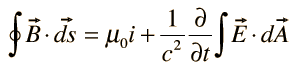Differential form Discussion
Index

Maxwell's equations concepts

 HyperPhysics***** Electricity and Magnetism R Nave
Go Back

# Maxwell's Equations

Differential form in the absence of magnetic or polarizable media:

 I. Gauss' law for electricity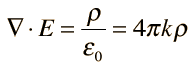II. Gauss' law for magnetism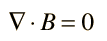III. Faraday's law of induction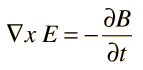IV. Ampere's law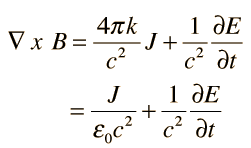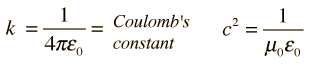Note: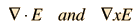here represent the vector operations divergence and curl, respectively.
 Integral form Discussion
 Differential form with magnetic and polarizable media
Index

Maxwell's equations concepts

 HyperPhysics***** Electricity and Magnetism R Nave
Go Back

# Maxwell's Equations

Differential form with magnetic and/or polarizable media:

 I. Gauss' law for electricity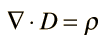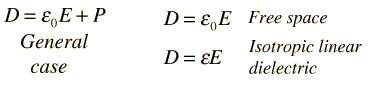II. Gauss' law for magnetismIII. Faraday's law of inductionIV. Ampere's law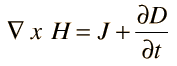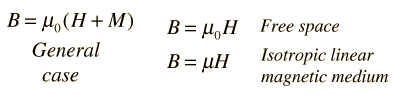Note:here represent the vector operations divergence and curl, respectively.
 Integral form Discussion
Index

Maxwell's equations concepts

 HyperPhysics***** Electricity and Magnetism R Nave
Go Back